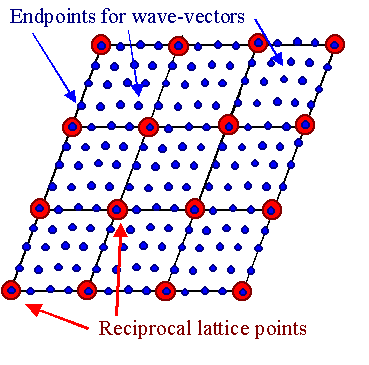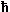# Fourier Transforms and Bloch's TheoremThe potential for the electron in a crystal lattice is periodic with the lattice, i.e. V(r + T) = V(r) with T = translation vector of the lattice. It is therefore always possible to develop V(r) into a Fourier series in reciprocal space and we obtain
V(r)  =  SG VG · exp (i · G ·r)The VG are the Fourier coefficients of the potential.G is a reciprocal lattice vector; the sum must be taken over all reciprocal vectors and there are infinitely many. We will, however, no longer use the underlining for vectors.In simple approximations it will be generally sufficient to consider only a few vectors of reciprocal space; i.e., most VG are 0.The wave function y(r) can also be Fourier transformed. Without loss of generality it can be expressed as a sum of the plane waves which are the solutions of the free electron gas problem (with V = 0):
y(r)  =  Sk Ck · exp (ikr)The Ck are the Fourier coefficients of the wave function and k denotes the wave vector as obtained from the simple free electron gas model (e.g. kx = ± nx · 2p/Lx).Since Lx must be a multiple of the lattice constant a, i.e. Lx = N · a (with N = Lx/a = number of elementary cells in Lx), all k-vectors can be written as
kx  = ±   nx · 2p
N · aWe know that 2p/a is simply the magnitude of the reciprocal lattice vector characterizing the set of planes perpenduclar to the direction of a (if taken as a unit vector of the elementary cell) with spacing a; i.e. for the {100} planes, nx · 2p/a gives the whole set of reciprocal lattice vectors with the same direction, and 1/N intersperses N points between the reciprocal lattice points defined by nx.In other words and generalized for three dimensions: The allowed points for k-vectors are points in reciprocal space interspersed between the lattice points of the reciprocal lattice.We have the following picture, with (for reasons of clarity, very few) blue k-points between the red G-points:The picture makes clear that any arbitrary wave vector k can be written as a sum of some reciprocal lattice vector G plus a suitable wave vector k'; i.e., we can always write k = G + k' and k' can always be confined to the 1. Brillouin zone (= the elementary cell of the reciprocal lattice).Alternatively, any reciprocal lattice vector G can always be written as G = kk' This is a relation that should look familiar; we are going to use it a few lines further down.If we now pluck both expressions into the Schrödinger equation
–2
2m
·   2y
r2
+  V(r)  =  E · y(r)and do the differentiations, we obtain
Sk  (· k)2
2m
· Ck · exp (ikr)  +  Sk' SG  Ck' · VG · exp (i · [k' + G] · r)  =  E · Sk Ck · exp (ik r)We have written k' in the double sum to indicate that it is not important how we sum up the components. That allows us to rename the summation indices and to replace k' as shown:
k' + G  =  k

k'  =  kGReshuffling the equation we obtain
Sk exp  ·  (·  – E (ik · r)  k)2 2m æ ç è æ è ö ø ö ÷ øIf this looks a bit like magic, you should consult the link.Since this equation holds for any space vector r, the expression in the red brackets must be zero by itself and we obtain

(·  –  E k)2 2m æ è ö øThis is nothing but Schrödinger's equation for crystals written as a collection of algebraic equations. It couples the Fourier coefficients VG of a periodic potential (which we know) to the Fourier coefficients Ck and Ck – G of the wave functions (which we want to calculate) in an unique way.If you have trouble visualizing this, write some parts of this infinite system of equations in a matrix as shown in the link for a slightly different situation.The problem now is much simplified. While our original Fourier expansion of the wave function was a sum containing a large number of coefficients Ck because we have a large number of k's, we now only have to consider a sum over G's - of which we have far less (if still infinitely many).This is so because our system of equations from above only contains Ck – G. Solving it, gives a definite wavefunction for any chosen k as a sum over just Ck – G coefficients.Since we can express all k vectors outside the 1st Brillouin zone as a sum of a k-vector in the first Brillouin zone and some G-vector (see above), we only have to consider N terms for the k-values.Since we have N k-vectors, we have N sets of equations, each one describing one wavefunction yk of which we now know that it can be expressed as a Fourier series over points in reciprocal space positioned at k – G with G = any reciprocal wave vector. This means we have
yk(r)  =  SG Ck – G · exp(i · [k – G] ·r)or, after rewriting the exponential
yk(r)  =  SG Ck – G · exp (–iGr) · exp (ikr)The first term shown in red, upon inspection, is nothing but the Fourier series of some function uk(r) that has the periodicity of the lattice; it is defined by:
uk(r)  =  SG Ck – G · exp (–iGr)We thus obtain

yk(r)  =  uk(r) · exp (–iGr)  =  SG Ck – G · exp (–iGr) · exp (ikr)And this is Bloch's theorem that we endeavored to prove.2.1.4 Periodic PotentialsBasic EquationsReciprocal LatticeDouble Sums and Index Shuffling

© H. Föll (Semiconductors - Script)